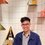# Daniel Liu's Hard Inequality

Hello every body.Sorry for too late.But this PROBLEM is very hard. In 1989,Jack Garfunkel recommended a inequality like this problem to Crux magazine in Canada.It's a hard problem and didn't have any solution at that time.

If we given $a,b,c$ are positive reals,the equality holds when $a=b=c$ and at that time,$k=\frac{\sqrt{3}}{\sqrt{2}}$

But $k=\frac{5}{4}$ must be better but we must let $a,b,c$ are non-negative and the equality holds when $a=b=3,c=0$ or it's permutation.

At present,there have many way to prove Jack Garfunkel's inequality. By applying full incremental variable,we have: $a+b+c+\frac{(a-b)(b-c)(a-c)(a+b+c)}{abc}\leq \frac{5\sqrt{2}}{4}\sqrt{x^2+y^2+z^2}$

Let $f(t)=\frac{(x+y+z-3t)^2}{(x-t)(y-t)(z-t)}$ is decreasing and $g(t)=\frac{5\sqrt{2}(x+y+z-3t)\sqrt{(x-t)^2+(y-t)^2+(z-t)^2}}{4}-(x+y+z-3t)^2$ is increasing in $[0;4]$. It's true because if we let $m=x-t;n=y-t,p=z-t$,$m,n,p > 0$ and $A=\sqrt{m^2+n^2+p^2};B=m+n+p$,we get: $m^2n^2p^2f'(t) \geq 0$ and $g'(t) \leq 0$. So that we get: $\frac{a}{\sqrt{a+b}}+\sqrt{b}\leq \frac{5}{4}\sqrt{a+b}$ This it's right because $RHS-LHS \geq 0$. The problem is solved. Another way is more interested:By C-S Inequality $LHS^2\leq \sum a(5a+b+9c)\sum \frac{a}{(a+b)(5a+b+9c)}$ We need to prove: $(a+b+c)\sum \frac{a}{(a+b)(5a+b+9c)}\leq \frac{5}{16}$ This inequality right because: $(RHS-LHS)(16\prod (5a+b+9c))=\sum ab(a+b)(a+9b)(a-3b)^2+243\sum a^3b^2c+835\sum a^3bc^2+232\sum a^4bc+1230a^2b^2c^2\geq 0$

The problem is solved.Note by Son Nguyen
5 years, 1 month ago

This discussion board is a place to discuss our Daily Challenges and the math and science related to those challenges. Explanations are more than just a solution — they should explain the steps and thinking strategies that you used to obtain the solution. Comments should further the discussion of math and science.

When posting on Brilliant:

• Use the emojis to react to an explanation, whether you're congratulating a job well done , or just really confused .
• Ask specific questions about the challenge or the steps in somebody's explanation. Well-posed questions can add a lot to the discussion, but posting "I don't understand!" doesn't help anyone.
• Try to contribute something new to the discussion, whether it is an extension, generalization or other idea related to the challenge.
• Stay on topic — we're all here to learn more about math and science, not to hear about your favorite get-rich-quick scheme or current world events.

MarkdownAppears as
*italics* or _italics_ italics
**bold** or __bold__ bold
- bulleted- list
• bulleted
• list
1. numbered2. list
1. numbered
2. list
Note: you must add a full line of space before and after lists for them to show up correctly
paragraph 1paragraph 2

paragraph 1

paragraph 2

[example link](https://brilliant.org)example link
> This is a quote
This is a quote
    # I indented these lines
# 4 spaces, and now they show
# up as a code block.

print "hello world"
# I indented these lines
# 4 spaces, and now they show
# up as a code block.

print "hello world"
MathAppears as
Remember to wrap math in $$ ... $$ or $ ... $ to ensure proper formatting.
2 \times 3 $2 \times 3$
2^{34} $2^{34}$
a_{i-1} $a_{i-1}$
\frac{2}{3} $\frac{2}{3}$
\sqrt{2} $\sqrt{2}$
\sum_{i=1}^3 $\sum_{i=1}^3$
\sin \theta $\sin \theta$
\boxed{123} $\boxed{123}$

## Comments

Sort by:

Top Newest

Can you explain this clearer?

1. What are you trying to describe?
2. What is the process to solve such a problem
3. How does someone come up with the various inequalities?

Staff - 5 years ago

Log in to reply

Ah yes, this was the SOS method you mentioned on the solutions page right? I believe it is from a China TST, as well.

- 5 years ago

Log in to reply

Also Vietnam TST

- 5 years ago

Log in to reply

Prove this Consider $a,b,c>0, abc=1$. Prove that

$\sum_{\mathrm{cyc}} \frac{a^{2}+b^{2}}{a^{8}+b^{8}}\leq a^{3}+b^{3}+c^{3}$

- 5 years, 1 month ago

Log in to reply

- 5 years, 1 month ago

Log in to reply

×

Problem Loading...

Note Loading...

Set Loading...